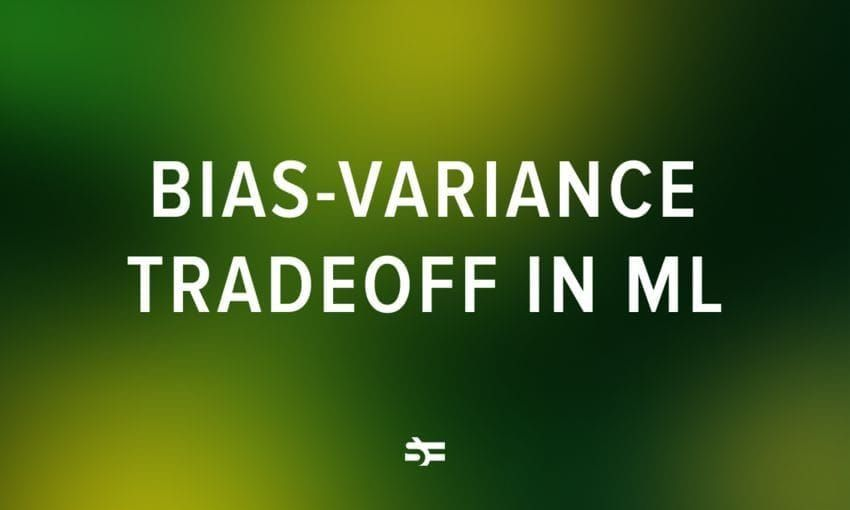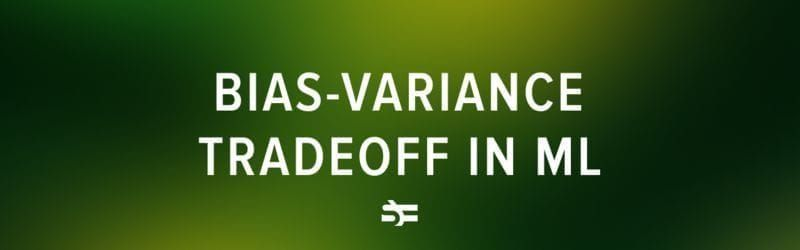# Naive Bayes Algorithm for Beginners

When you start learning about artificial intelligence, one of the first things that you encounter is Naive Bayes algorithms. Why are Naive Bayes classifiers so fundamental to AI and ML? Let’s find out.

## Uncertainty and probability in machine learning

Artificial intelligence has to operate with data that in many cases is big but, regardless, incomplete. Just like humans, the computer has to take risks and think about the future that is not certain.

Uncertainty is hard to bear for human beings. But in machine learning, there are certain algorithms that help to find your way around this limitation. The Naive Bayes machine learning algorithm is one of the tools to deal with uncertainty with the help of probabilistic methods.

Probability is a field of math that enables us to reason about uncertainty and assess the likelihood of some results or events. When you work with predictive ML modeling, you have to predict uncertain future. For example, you may try to predict the performance of an Olympic champion during the next Olympics based on past results. Even if they won before, it doesn’t mean they will win this time. Unpredictable factors such as an argument with their partner this morning or no time to have breakfast may or may not influence their results.

Therefore, uncertainty is integral to machine learning modeling since, well, life is complicated and nothing is perfect. Three main sources of uncertainty in machine learning are noisy data, incomplete coverage of the problem, and imperfect models.

## Computing probability

In machine learning, we are interested in conditional probabilities. We are interested not in the general probability that something will happen, but the likelihood it will happen given that something else happens. For example, by using conditional probability, we can try to answer the question of what is the probability (P) that an athlete wins the race given their results for the past 10 years.

This is how conditional probability is defined: the probability of a, given b = the joint probability of both a and b happening, divided by the probability of b.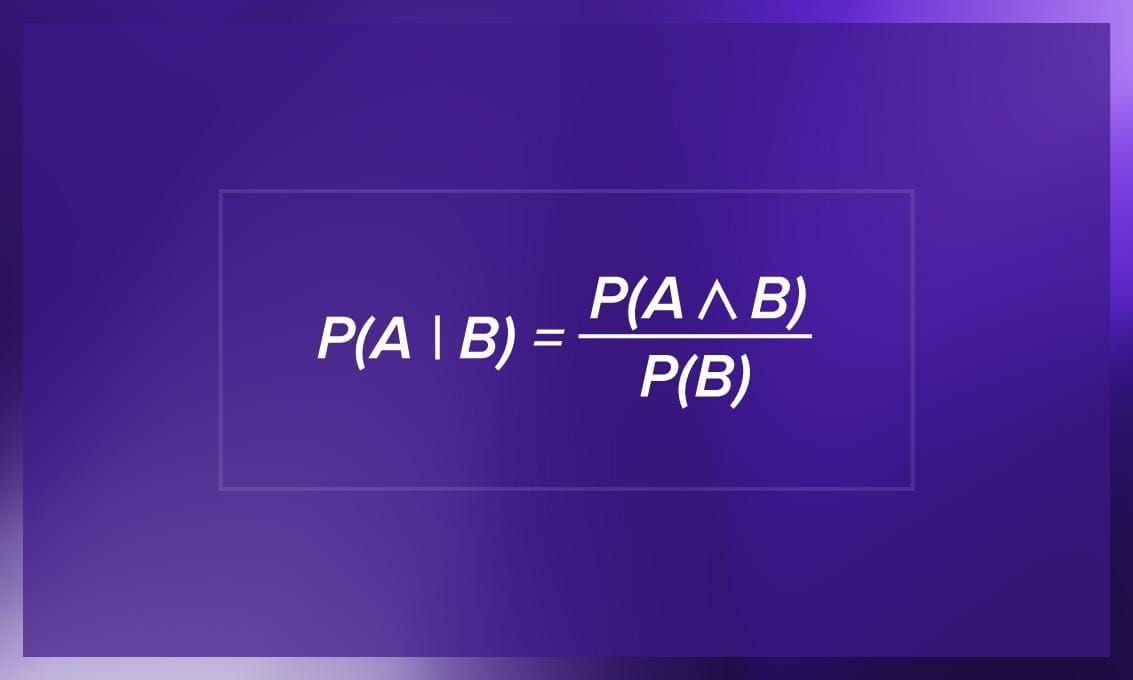By juggling with the conditional probability equation a little bit, you can come to another equation, which is better known as the Bayes’ theorem.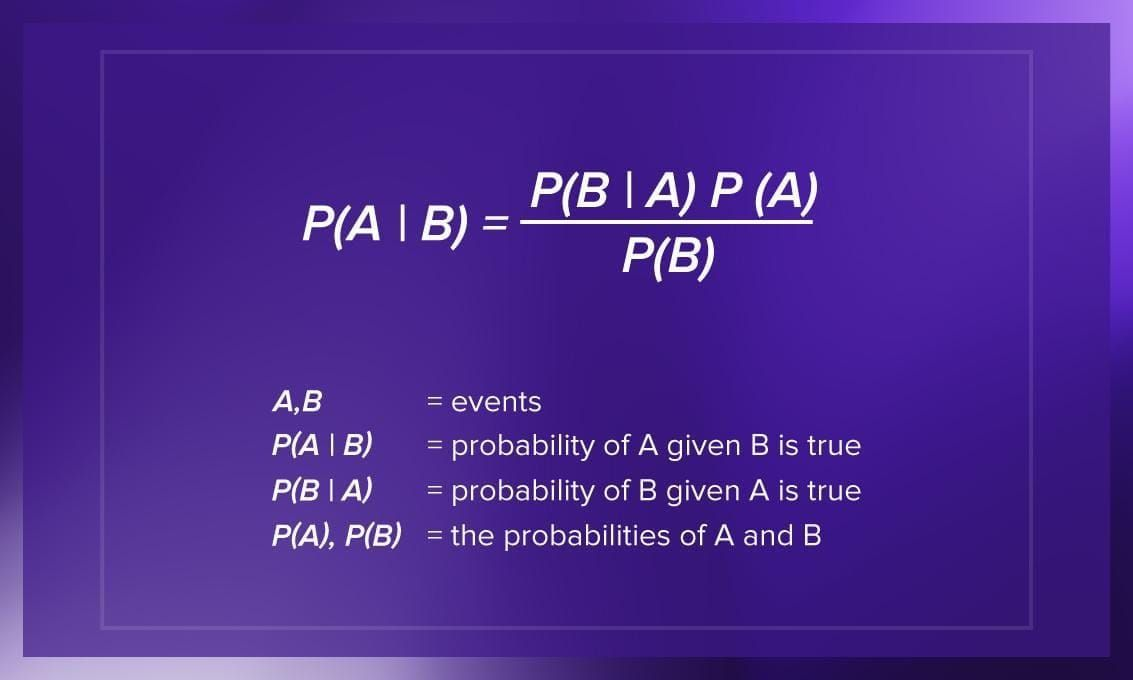## Bayesian probability

Bayes’ theorem allows us to calculate conditional probabilities. It comes extremely handy because it enables us to use some knowledge that we already have (called prior) to calculate the probability of a related event. It is used in developing models for classification and predictive modeling problems such as Naive Bayes.

Bayes’ rule is commonly used in probability theory to compute the conditional probability.

For example, let’s say we are consulting an athlete on pre-game diet and she presents this data:

• 80% of the time, if she wins the race, she had a good breakfast. This is P(breakfast|win).
• 60% of the time, she has a nice breakfast P(breakfast). This is our b.
• 20% of the time, she wins a race P(win). This is our a.

By applying Bayes’ rule, we can compute P(win|breakfast) to be 0.2 times 0.8, divided by 0.6 = 0.26. That is, the probability that she wins the race given a hearty breakfast is 26%. Clearly, an extra load of carbs gives her endurance throughout the competition.

What is important is that we can not only discover how evidence impacts the probability of an event, but by how much.

## Naive Bayes

Naive Bayes is a simple supervised machine learning algorithm that uses the Bayes’ theorem with strong independence assumptions between the features to procure results. That means that the algorithm just assumes that each input variable is independent. It really is a naive assumption to make about real-world data. For example, if you use Naive Bayes for sentiment analysis, given the sentence ‘I like Harry Potter’, the algorithm will look at the individual words and not the full sentence. In a sentence, words that stand next to each other influence the meaning of each other, and the position of words in a sentence is also important. However, for the algorithm, phrases like ‘I like Harry Potter’, Harry Potter like I’, and ‘Potter I like Harry’ are the same.

Turns out that the algorithm is able to effectively solve many complex problems. For example, building a text classifier with Naive Bayes is much easier than with more hyped algorithms such as neural networks. The model works well even with insufficient or mislabeled data, so you don’t have to ‘feed’ it hundreds of thousands of examples before you can get something reasonable out of it. Even if Naive Bayes can take as much as 50 lines, it is very effective.

As for the weaker points of Naive Bayes, it performs better with categorical than with numerical values. It automatically assumes the bell curve distribution, which is not always right. Also, if a categorical variable has a category in the test data set that wasn’t included in the training data set, then the model will assign it a 0 probability and will be unable to make a prediction. This is called the Zero Frequency problem.

To solve this, we will have to use the smoothing technique. And, of course, its main disadvantage is that in real life it’s rare that events are completely independent. You have to apply other algorithms to track causal dependency.

If you would like to get started with programming your first Naive Classifier, I recommend this name-gender classifier. The notebook guides you through the process step by step and provides you with the necessary data to train and test your model.

## Types of Naive Bayes classifiers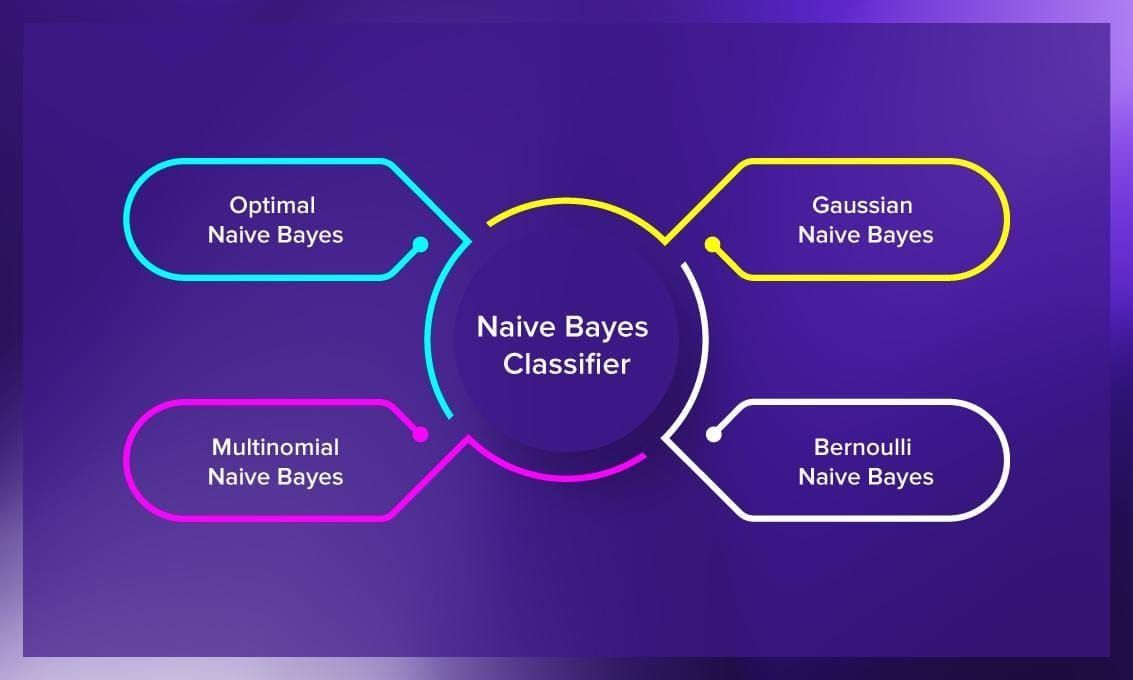There are several types of Naive Bayes.

### Optimal Naive Bayes

This classifier chooses the class that has the greatest a posteriori probability of occurrence (so called maximum a posteriori estimation, or MAP). As follows from the name, it really is optimal but going through all possible options is rather slow and time-consuming.

### Gaussian Naive Bayes

Gaussian Bayes is based on Gaussian, or normal distribution. It significantly speeds up the search and, under some non-strict conditions, the error is only two times higher than in Optimal Bayes (that’s good!).

### Multinomial Naive Bayes

It is usually applied to document classification problems. It bases its decisions on discrete features (integers), for example, on the frequency of the words present in the document.

### Bernoulli Naive Bayes

Bernoulli is similar to the previous type but the predictors are boolean variables. Therefore, the parameters used to predict the class variable can only have yes or no values, for example, if a word occurs in the text or not.

## Where the Naive Bayes algorithm can be used

Here are some of the common applications of Naive Bayes for real-life tasks:

• Document classification. This algorithm can help you to determine to which category a given document belongs. It can be used to classify texts into different languages, genres, or topics (through the presence of keywords).
• Spam filtering. Naive Bayes easily sorts out spam using keywords. For example, in spam, you can see the word ‘viagra’ much more often than in regular mail. The algorithm must be trained to recognize such probabilities and, then, it can efficiently apply them for spam filtering.
• Sentiment analysis. Based on what emotions the words in a text express, Naive Bayes can calculate the probability of it being positive or negative. For example, in customer reviews, ‘good’ or ‘inexpensive’ usually mean that the customer is satisfied. However, Naive Bayes is not sensitive to sarcasm.
• Image classification. For personal and research purposes, it is easy to build a Naive Bayesian classifier. It can be trained to recognize hand-written digits or put images into categories through supervised machine learning.

## Bayesian poisoning

Bayesian poisoning is a technique used by email spammers to try to reduce the effectiveness of spam filters that use Bayes’ rule. They hope to increase the rate of false positives of the spam filter by turning previously innocent words into spam words in a Bayesian database. Adding words that were more likely to appear in non-spam emails is effective against a naive Bayesian filter and allows spam to slip through. However, retraining the filter effectively prevents all types of attacks. That is why Naive Bayes is still being used for spam detection, along with certain heuristics such as blacklist.

## Final words

Looking forward to reading more materials about machine learning? We’re already working on it! In the meantime, you can explore some of the articles in our AI and ML sections:

If you are looking for AI and machine learning services, our expert team will be happy to develop a unique solution for your business. Contact us today.More from Serokell